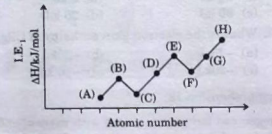#In the above graph, element F is

1. A

oxygen

2. B

nitrogen

3. C

fluorine

4. D

neon

FREE Lve Classes, PDFs, Solved Questions, PYQ's, Mock Tests, Practice Tests, and Test Series!

+91

Verify OTP Code (required)

I agree to the terms and conditions and privacy policy.

### Solution:

In the above graph, element F is oxygen. The alphabet represents as,

$\begin{array}{l}\mathrm{A}\to \mathrm{Li} \mathrm{B}\to \mathrm{Be}\\ \mathrm{C}\to \mathrm{B} \mathrm{D}\to \mathrm{C}\\ \mathrm{E}\to \mathrm{N} \mathrm{F}\to \mathrm{O}\\ \mathrm{G}\to \mathrm{F} \mathrm{H}\to \mathrm{Ne}\end{array}$

Hence, option A is correct.

## Related content

Join Infinity Learn Regular Class Program!

Sign up & Get instant access to FREE PDF's, solved questions, Previous Year Papers, Quizzes and Puzzles!

+91

Verify OTP Code (required)

I agree to the terms and conditions and privacy policy.